Courses

# Test: Probability- 2

## 10 Questions MCQ Test IBPS Clerk Prelims - Study Material, Mock Tests | Test: Probability- 2

Description
This mock test of Test: Probability- 2 for Quant helps you for every Quant entrance exam. This contains 10 Multiple Choice Questions for Quant Test: Probability- 2 (mcq) to study with solutions a complete question bank. The solved questions answers in this Test: Probability- 2 quiz give you a good mix of easy questions and tough questions. Quant students definitely take this Test: Probability- 2 exercise for a better result in the exam. You can find other Test: Probability- 2 extra questions, long questions & short questions for Quant on EduRev as well by searching above.
QUESTION: 1

### What is the probability of selecting a prime number from 1,2,3,... 10 ?

Solution:

Total count of numbers, n(S) = 10

Prime numbers in the given range are 2,3,5 and 7
Hence, total count of prime numbers in the given range, n(E) = 4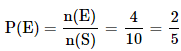QUESTION: 2

### 3 balls are drawn randomly from a bag contains 3 black, 5 red and 4 blue balls. What is the probability that the balls drawn contain balls of different colors?

Solution:

Total number of balls = 3 + 5 + 4 = 12

Let S be the sample space.
n(S) = Total number of ways of drawing 3 balls out of 12 = 12C3

Let E = Event of drawing 3 different coloured balls

To get 3 different coloured balls,we need to select one black ball from 3 black balls,
one red ball from 5 red balls, one blue ball from 4 blue balls

Number of ways in which this can be done = 3C1 × 5C1 × 4C1
i.e., n(E) = 3C1 × 5C1 × 4C1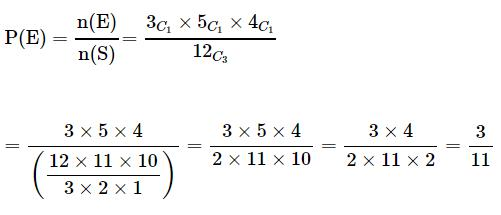QUESTION: 3

### 5 coins are tossed together. What is the probability of getting exactly 2 heads?

Solution:

Total number of outcomes possible when a coin is tossed = 2 (? Head or Tail)
Hence, total number of outcomes possible when 5 coins are tossed, n(S) = 25

E = Event of getting exactly 2 heads when 5 coins are tossed
n(E) = Number of ways of getting exactly 2 heads when 5 coins are tossed = 5C2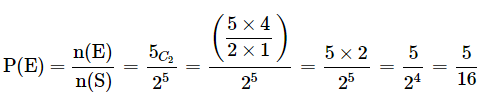QUESTION: 4

What is the probability of drawing a "Queen" from a deck of 52 cards?

Solution:

Total number of cards, n(S) = 52

Total number of "Queen" cards, n(E) = 4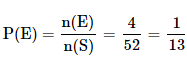QUESTION: 5

A card is randomly drawn from a deck of 52 cards. What is the probability getting an Ace or King or Queen?

Solution:

Total number of cards = 52

Total number of Ace cards = 4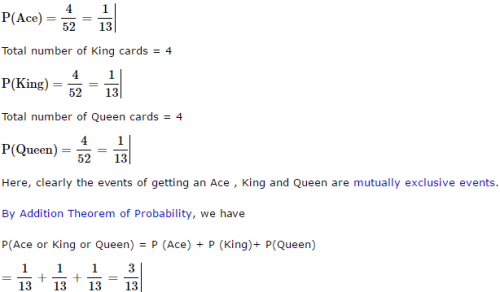QUESTION: 6

A card is randomly drawn from a deck of 52 cards. What is the probability getting a five of Spade or Club?

Solution: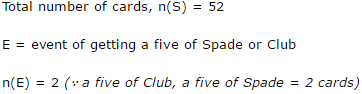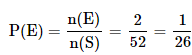QUESTION: 7

When two dice are rolled, what is the probability that the sum is either 7 or 11?

Solution:

Total number of outcomes possible when a die is rolled = 6 (? any one face out of the 6 faces)

Hence, total number of outcomes possible when two dice are rolled = 6 × 6 = 36

To get a sum of 7, the following are the favourable cases.
(1, 6), (2, 5), {3, 4}, (4, 3), (5, 2), (6,1)

=> Number of ways in which we get a sum of 7 = 6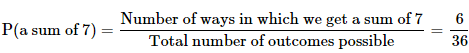To get a sum of 11, the following are the favourable cases. (5, 6), (6, 5) => Number of ways in which we get a sum of 11 = 2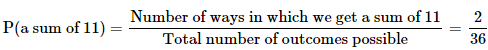Here, clearly the events are mutually exclusive events. By Addition Theorem of Probability, we have P(a sum of 7 or a sum of 11) = P(a sum of 7) + P( a sum of 11)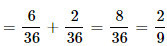QUESTION: 8

A card is randomly drawn from a deck of 52 cards. What is the probability getting either a King or a Diamond?

Solution:

Total number of cards = 52

Total Number of King Cards = 4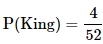Total Number of Diamond Cards = 13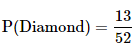Total Number of Cards which are both King and Diamond = 1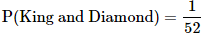Here a card can be both a Diamond card and a King. Hence these are not mutually exclusive events. (Reference : mutually exclusive events) . By Addition Theorem of Probability, we have P(King or a Diamond) = P(King) + P(Diamond) – P(King and Diamond)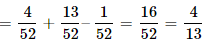QUESTION: 9

John and Dani go for an interview for two vacancies. The probability for the selection of John is 1/3 and whereas the probability for the selection of Dani is 1/5. What is the probability that none of them are selected?

Solution: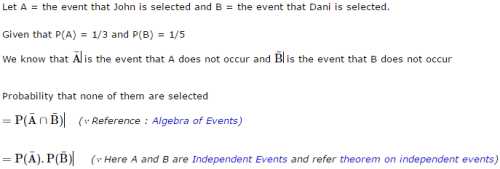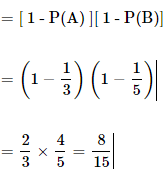QUESTION: 10

John and Dani go for an interview for two vacancies. The probability for the selection of John is 1/3 and whereas the probability for the selection of Dani is 1/5. What is the probability that only one of them is selected?

Solution: